Ex 7.4

Chapter 7 Class 6 Fractions
Serial order wise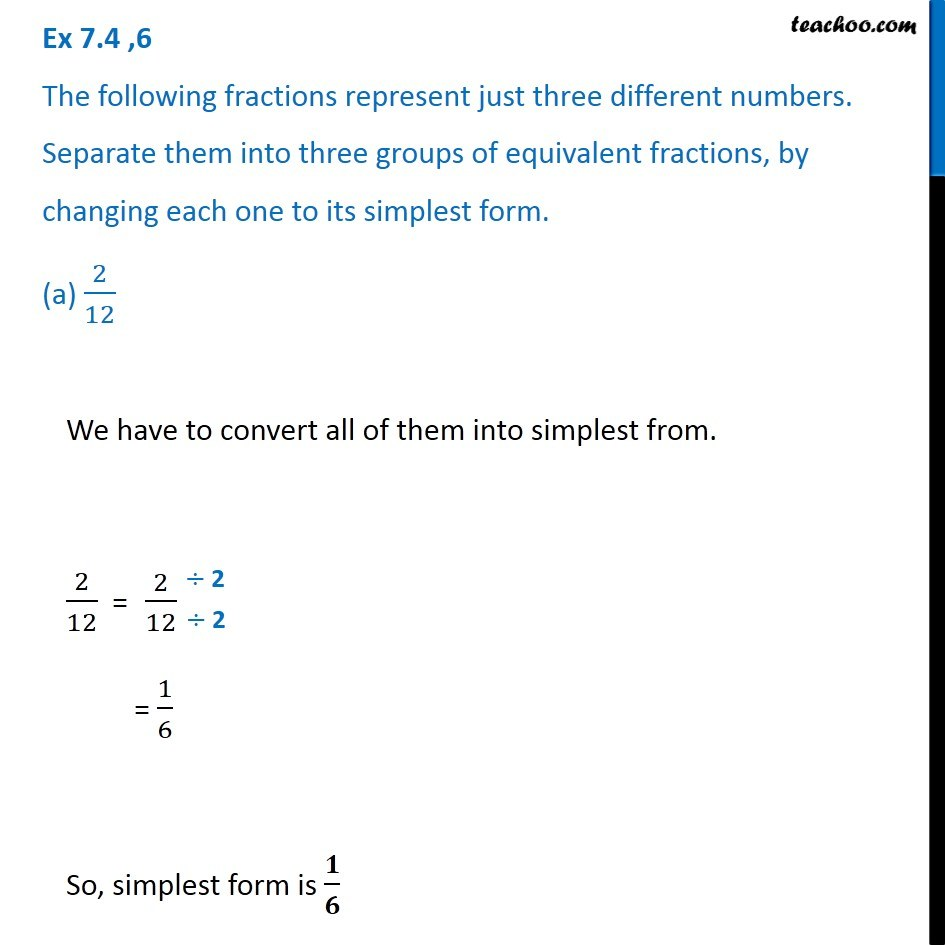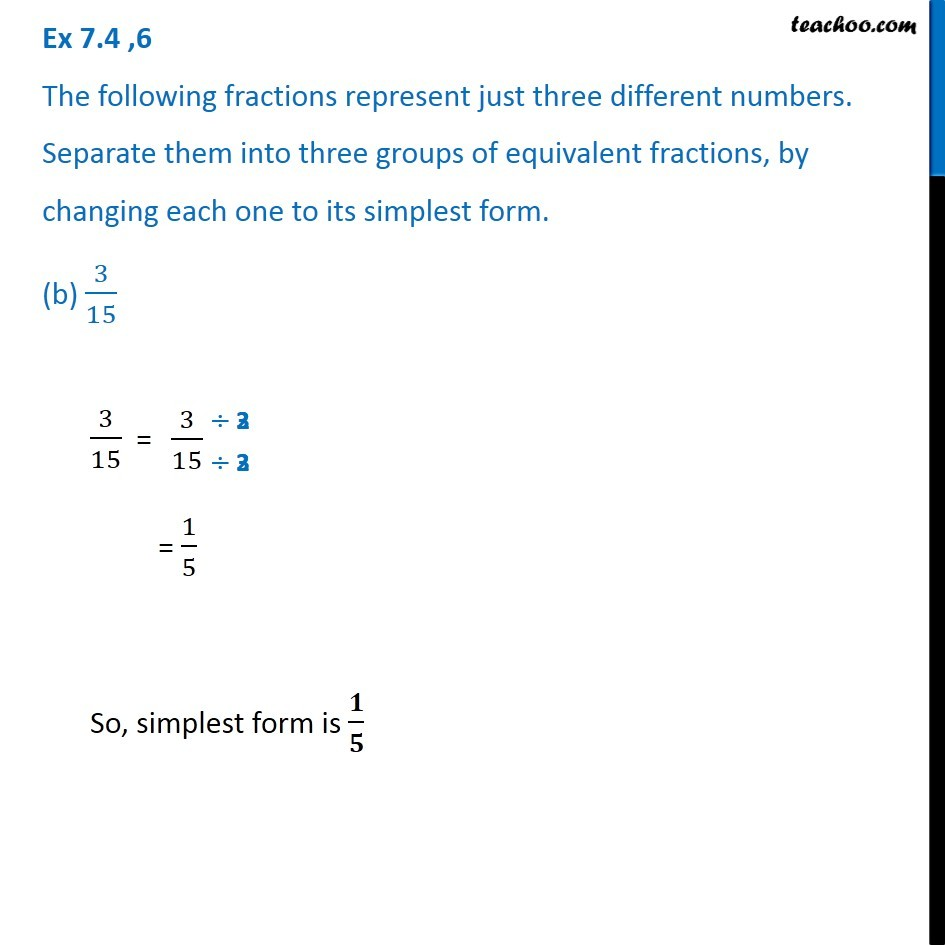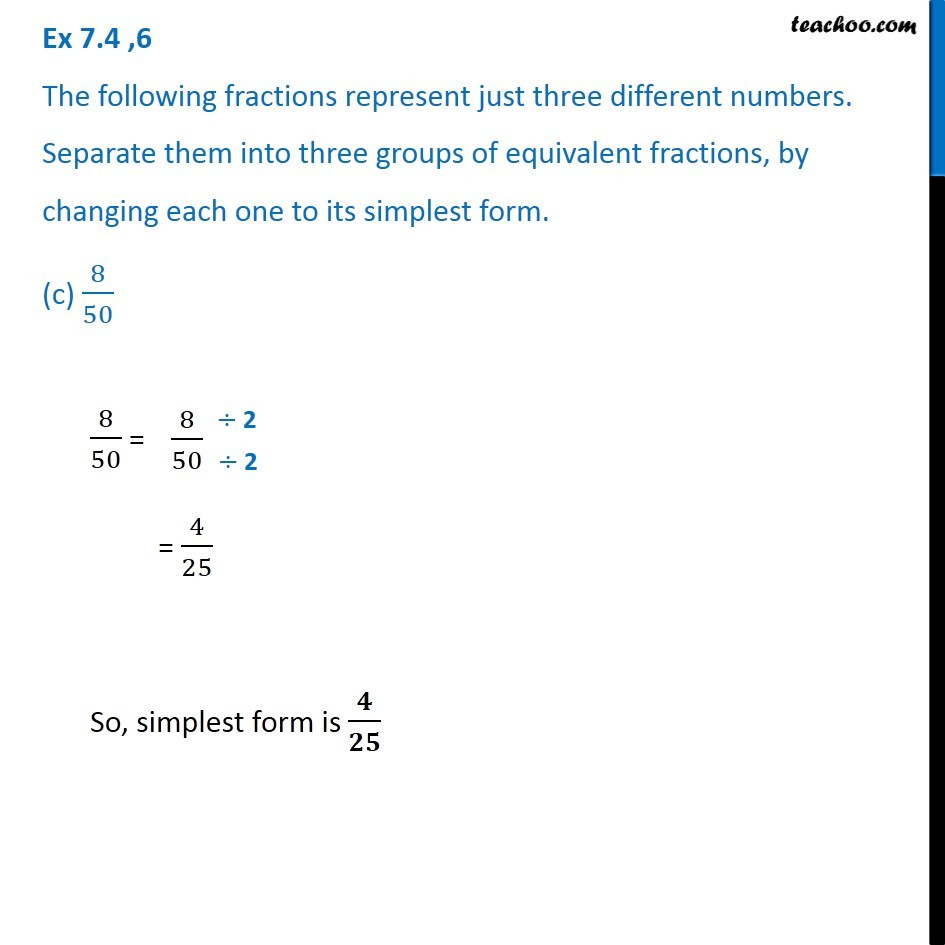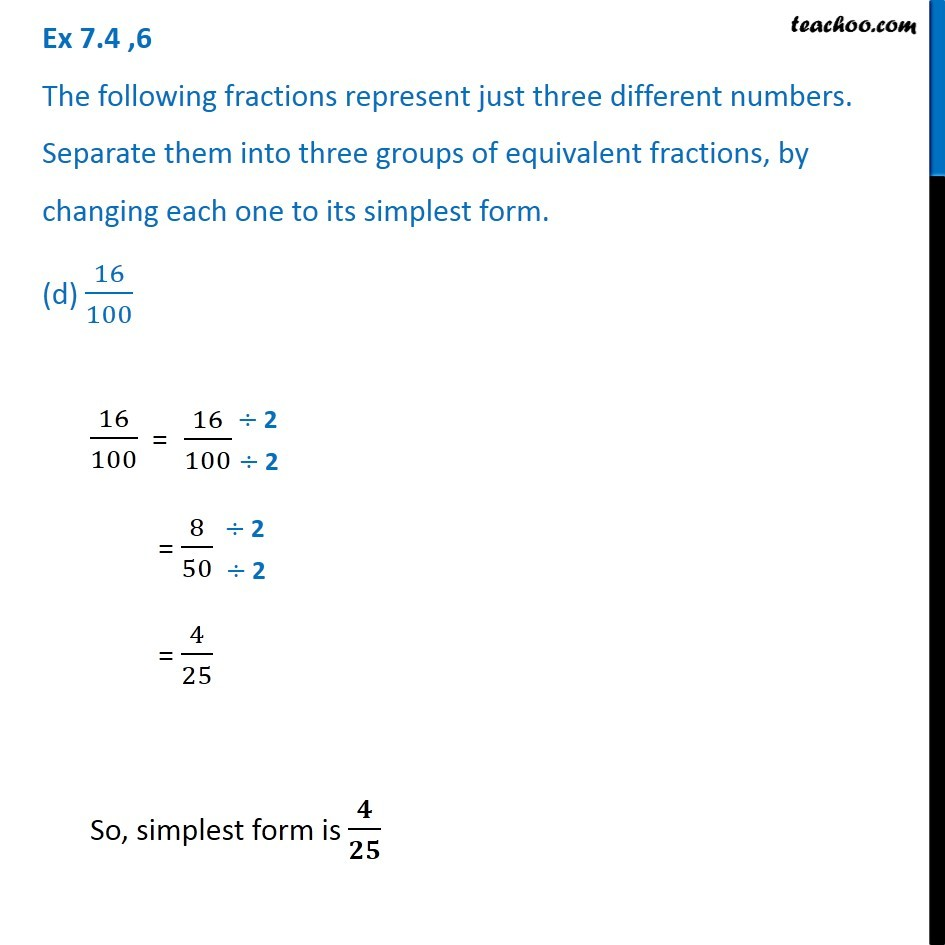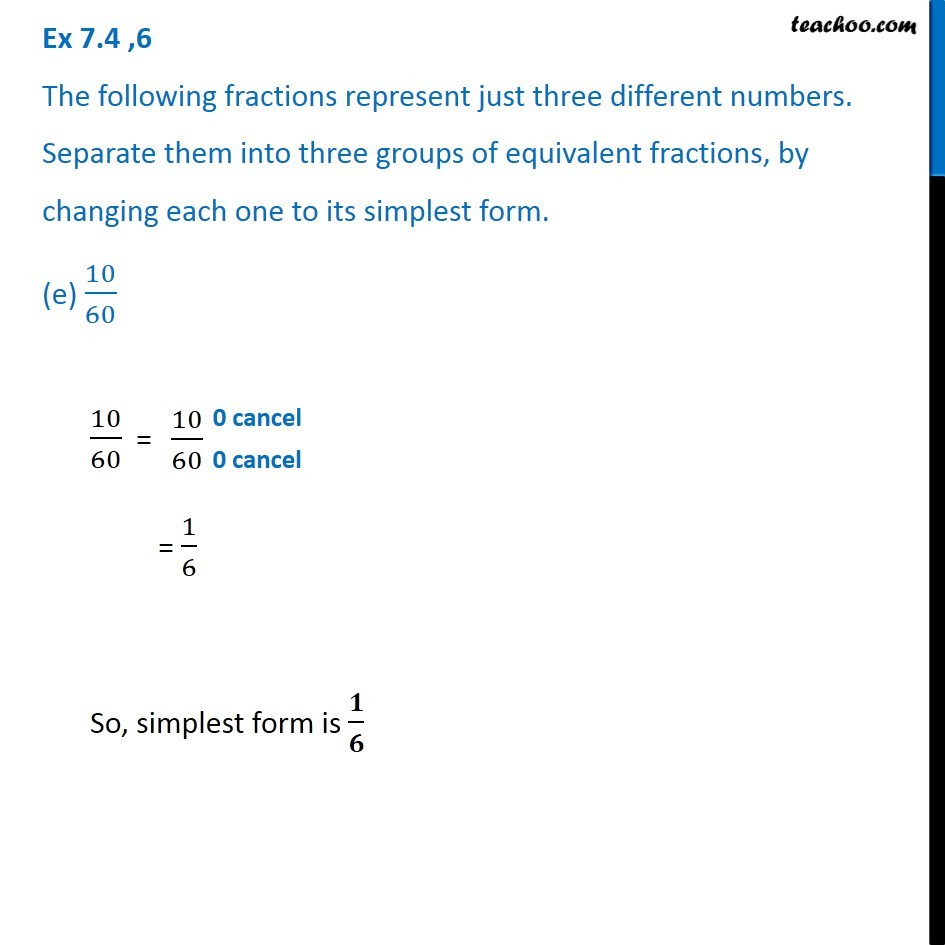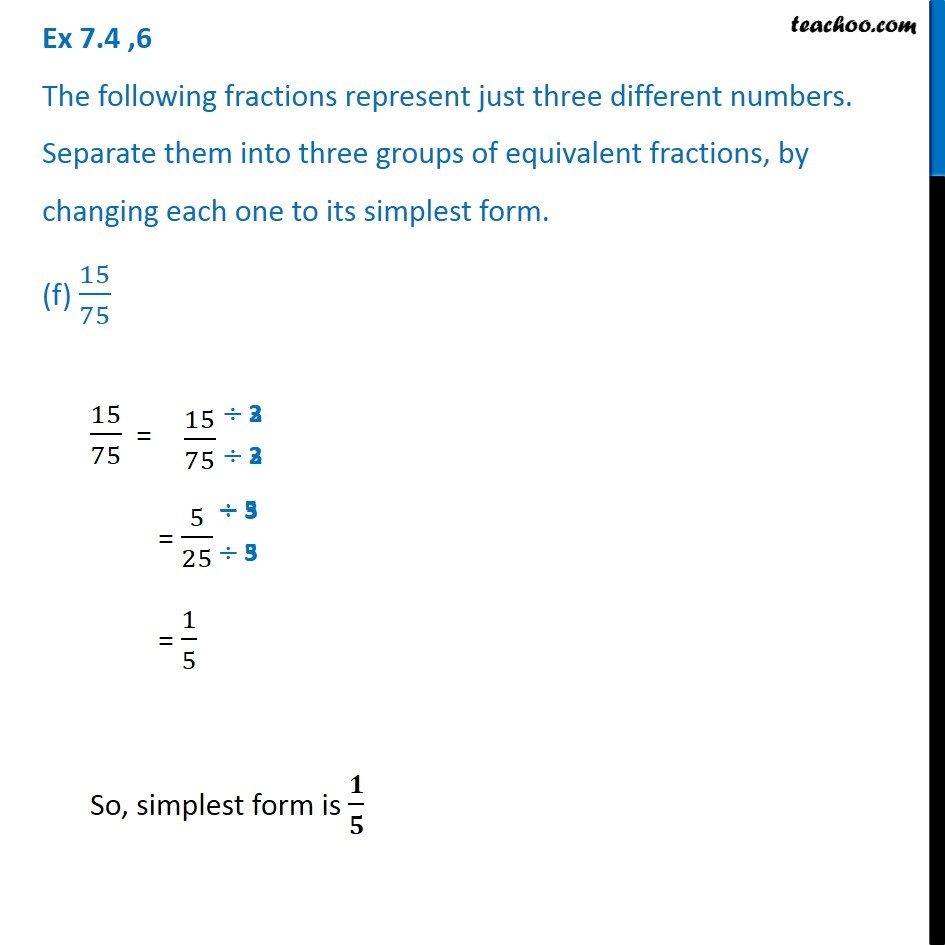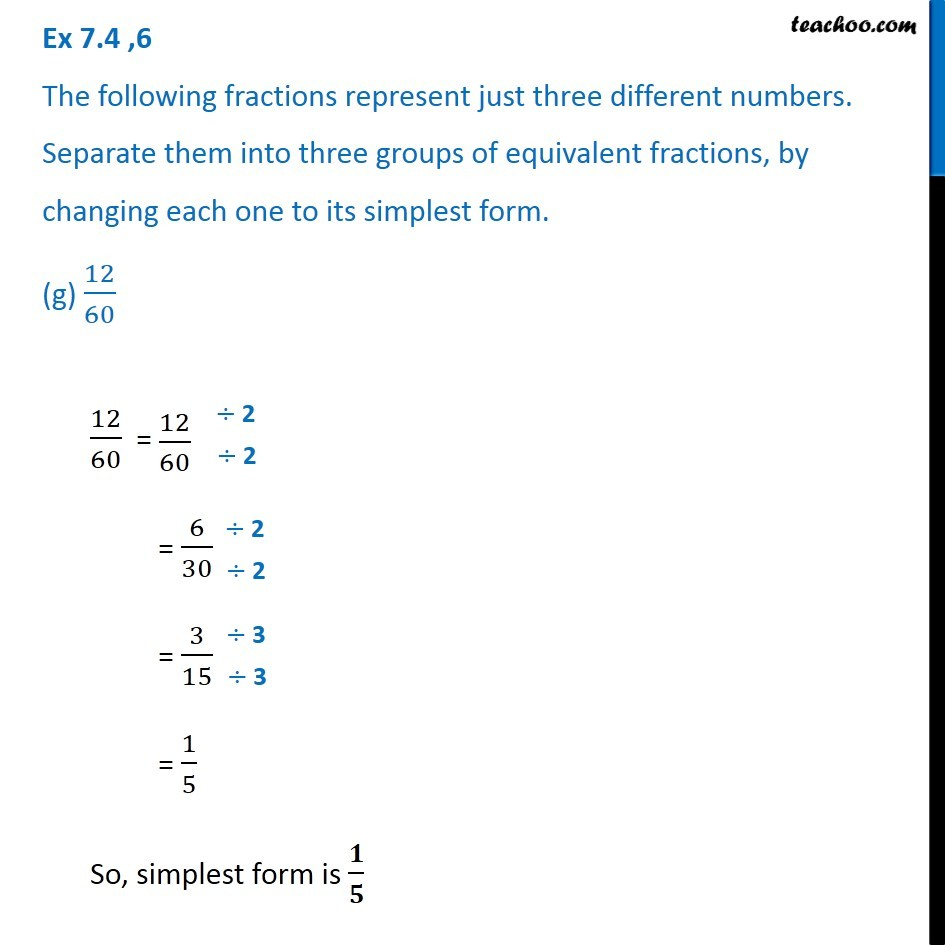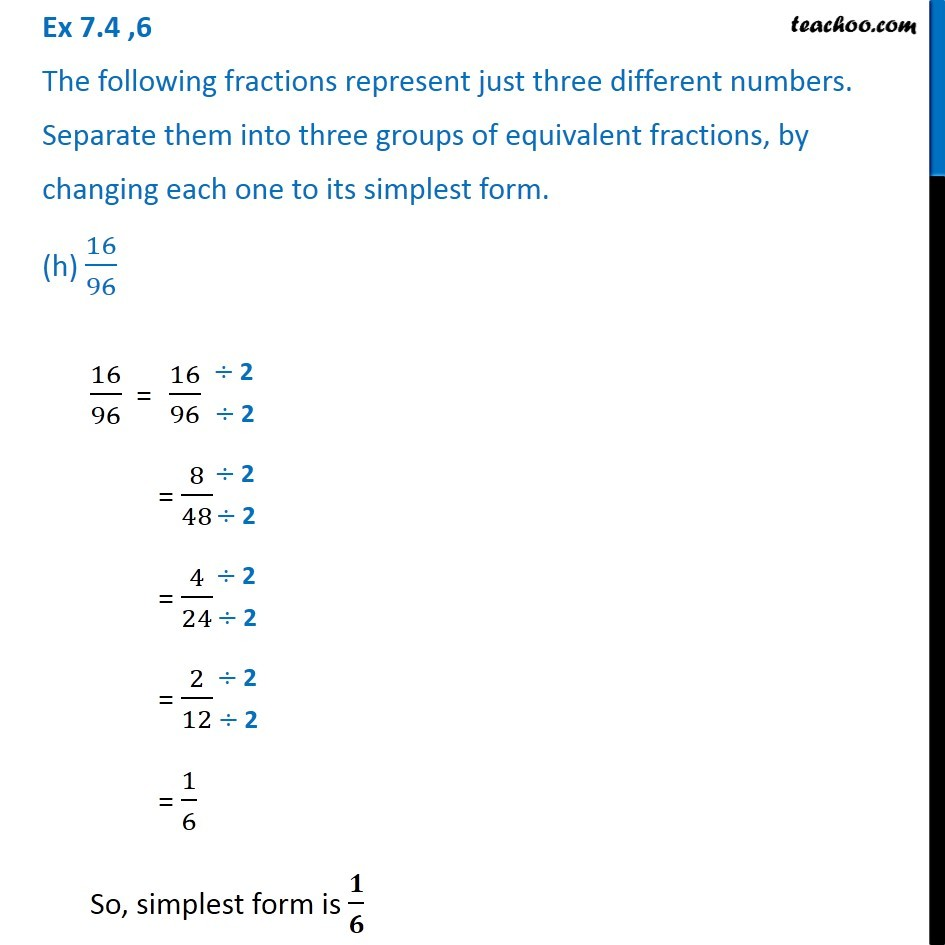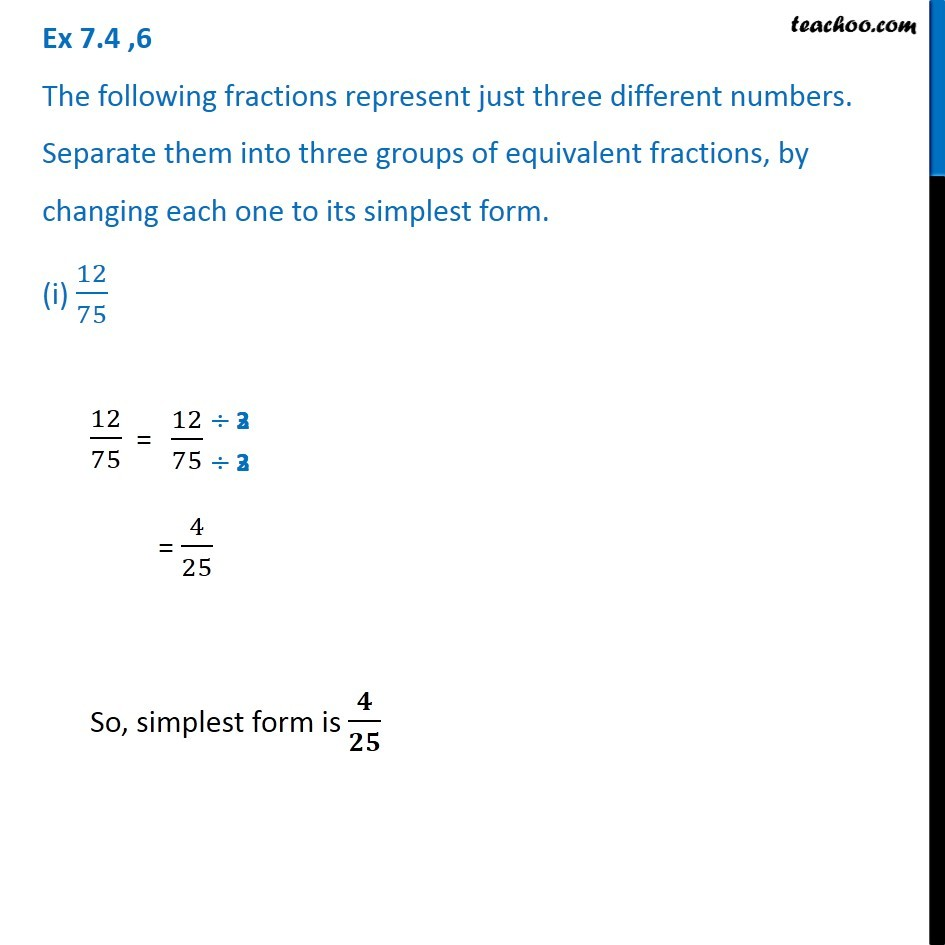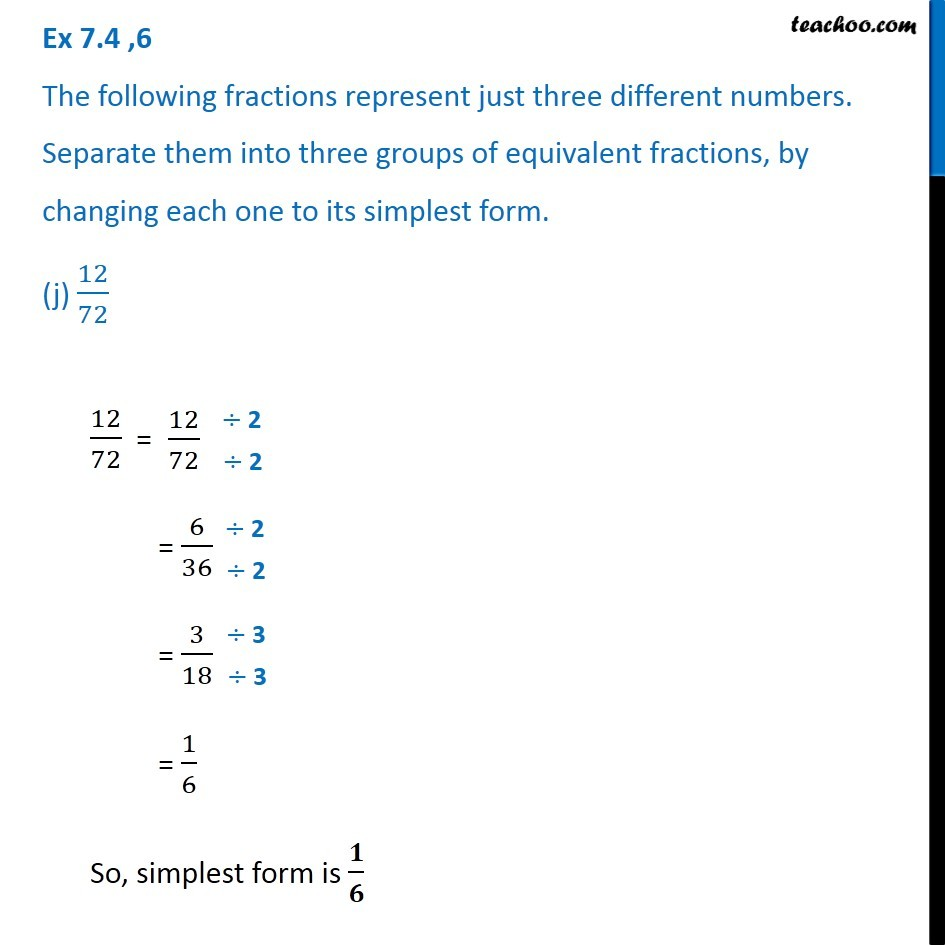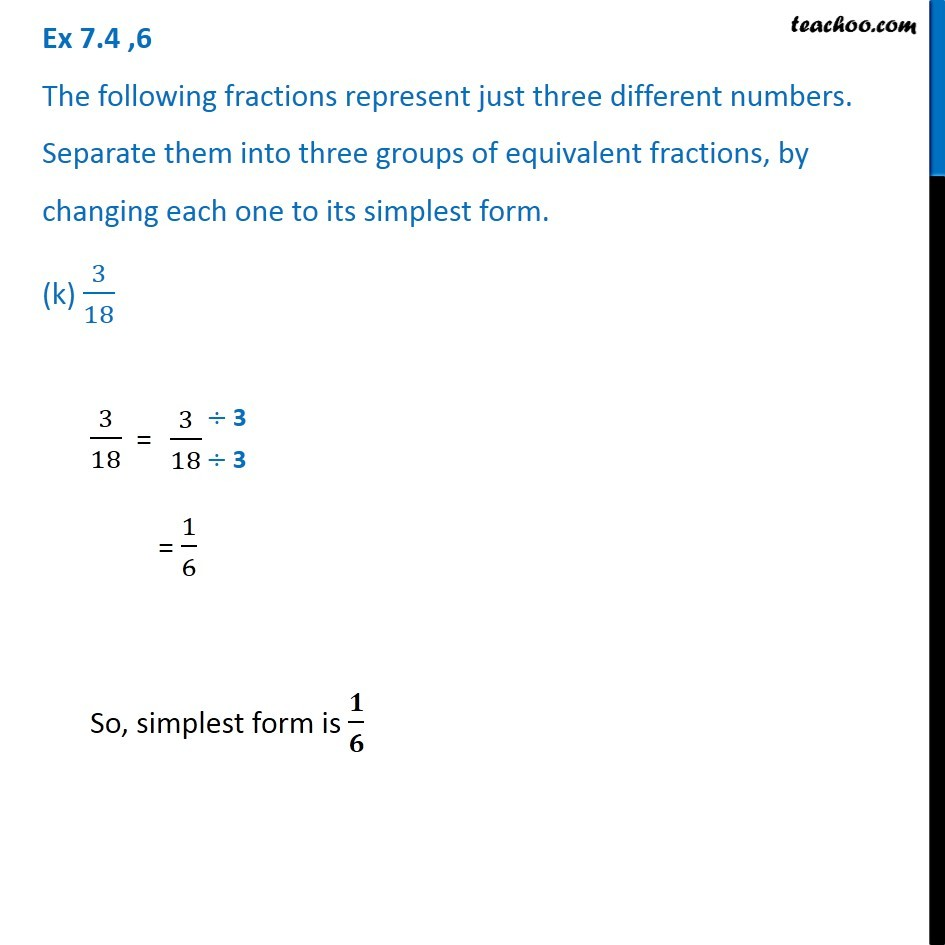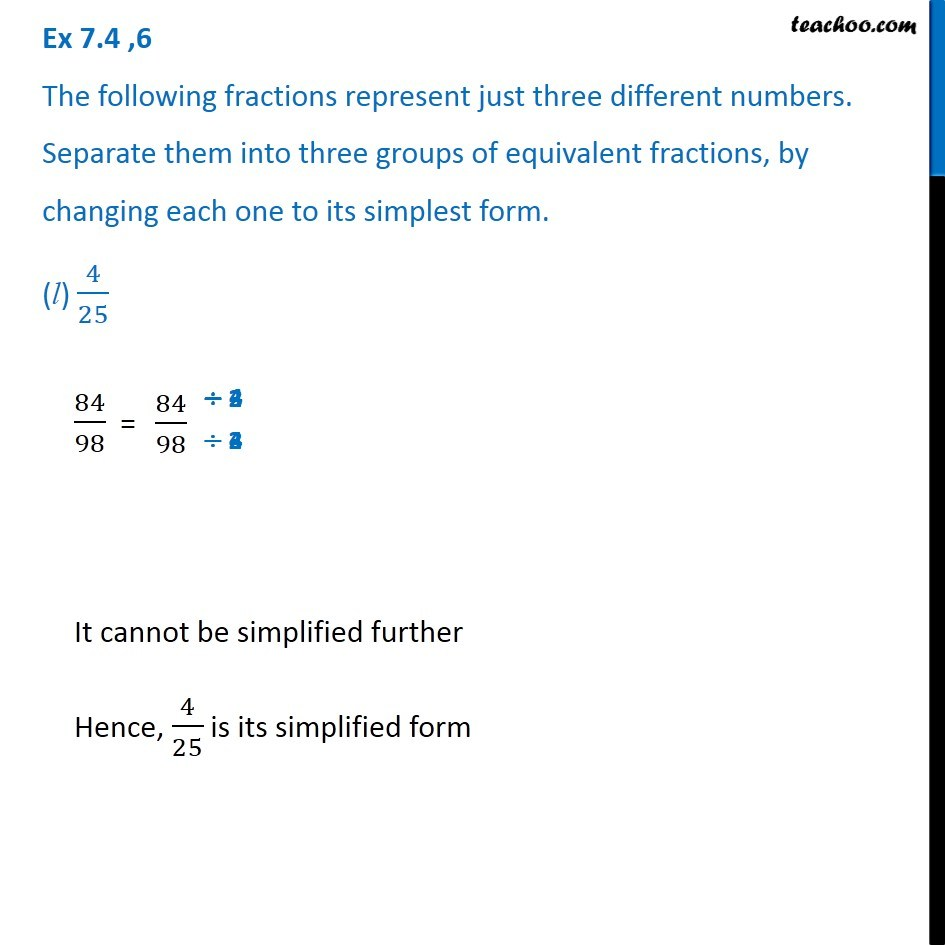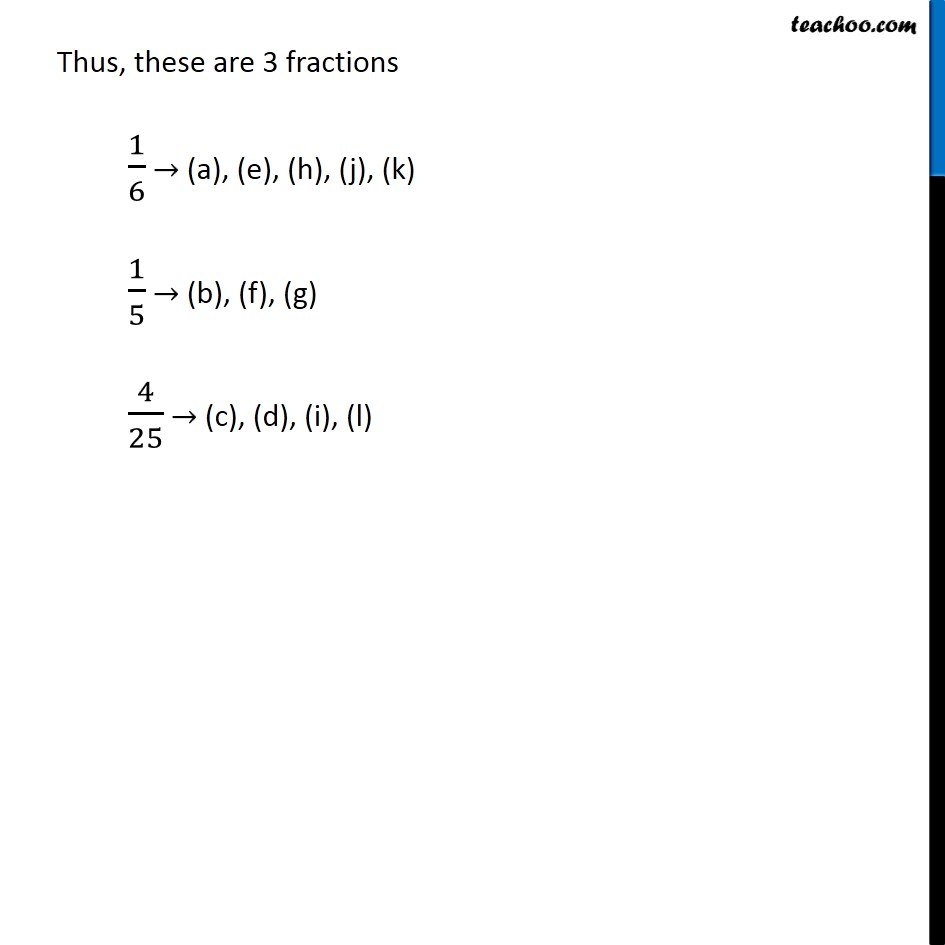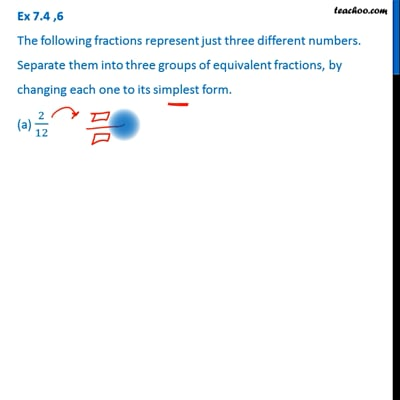This video is only available for Teachoo black users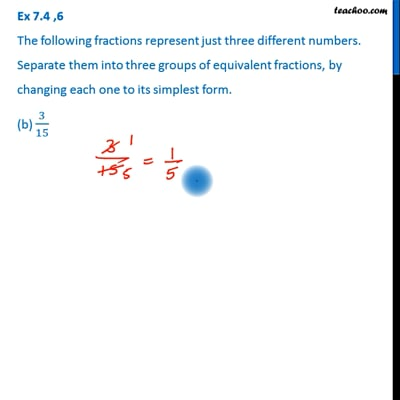This video is only available for Teachoo black users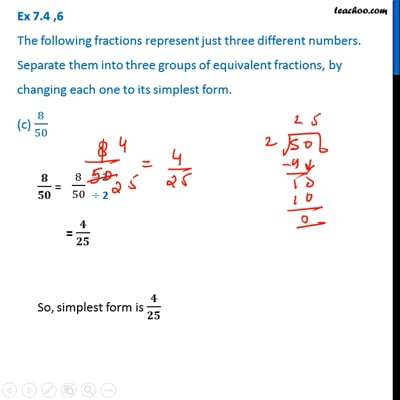This video is only available for Teachoo black users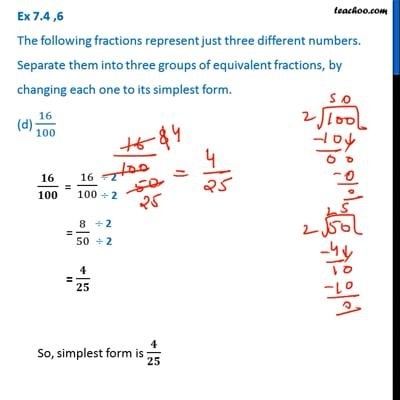This video is only available for Teachoo black users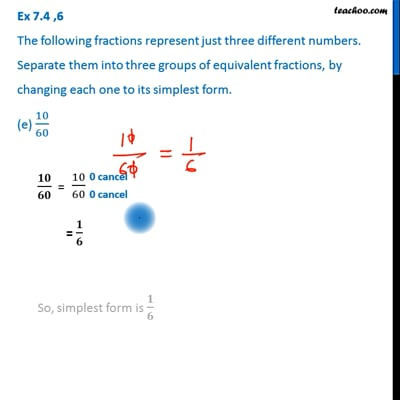This video is only available for Teachoo black users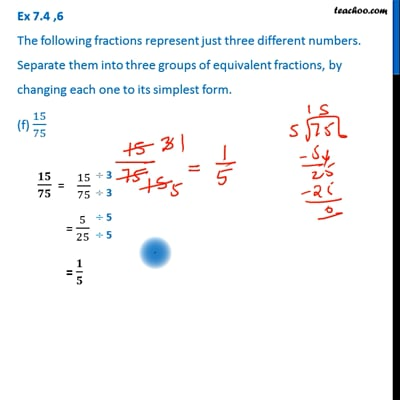This video is only available for Teachoo black users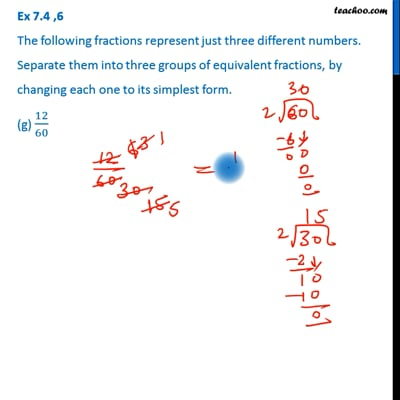This video is only available for Teachoo black usersThis video is only available for Teachoo black users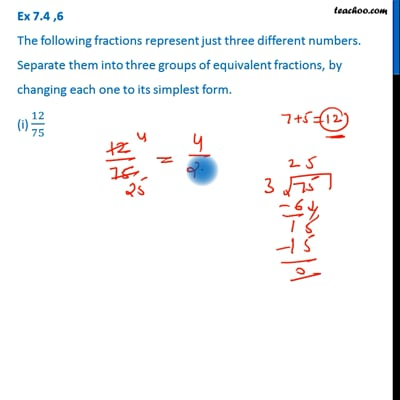This video is only available for Teachoo black users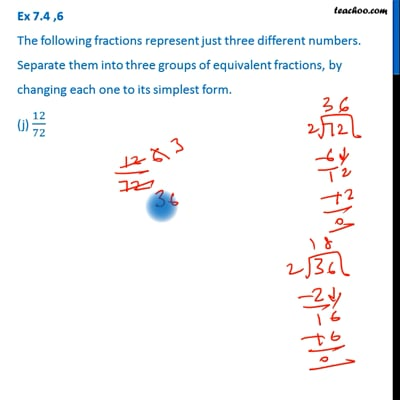This video is only available for Teachoo black users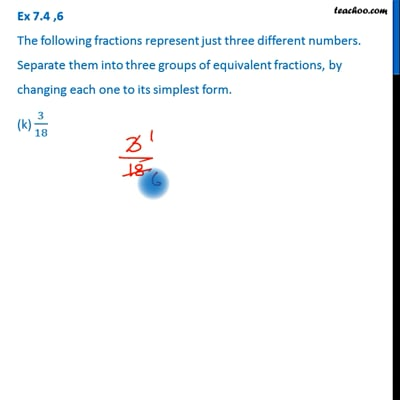This video is only available for Teachoo black users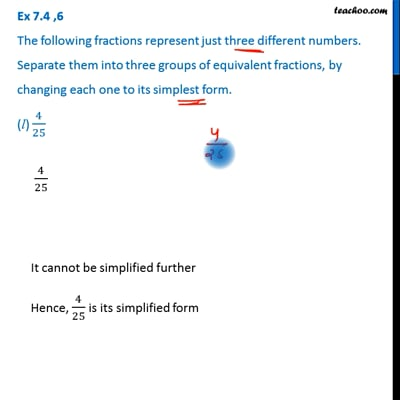This video is only available for Teachoo black users

Get live Maths 1-on-1 Classs - Class 6 to 12

### Transcript

Ex 7.4 ,6 The following fractions represent just three different numbers. Separate them into three groups of equivalent fractions, by changing each one to its simplest form. (a) 2/12 We have to convert all of them into simplest from. 2/12 = = 1/6 So, simplest form is 𝟏/𝟔 Ex 7.4 ,6 The following fractions represent just three different numbers. Separate them into three groups of equivalent fractions, by changing each one to its simplest form. (b) 3/15 3/15 = = 1/5 So, simplest form is 𝟏/𝟓 Ex 7.4 ,6 The following fractions represent just three different numbers. Separate them into three groups of equivalent fractions, by changing each one to its simplest form. (c) 8/50 8/50 = = 4/25 So, simplest form is 𝟒/𝟐𝟓 Ex 7.4 ,6 The following fractions represent just three different numbers. Separate them into three groups of equivalent fractions, by changing each one to its simplest form. (d) 16/100 16/100 = = 8/50 = 4/25 So, simplest form is 𝟒/𝟐𝟓 Ex 7.4 ,6 The following fractions represent just three different numbers. Separate them into three groups of equivalent fractions, by changing each one to its simplest form. (e) 10/60 10/60 = = 1/6 So, simplest form is 𝟏/𝟔 Ex 7.4 ,6 The following fractions represent just three different numbers. Separate them into three groups of equivalent fractions, by changing each one to its simplest form. (f) 15/75 15/75 = = 5/25 = 1/5 So, simplest form is 𝟏/𝟓 Ex 7.4 ,6 The following fractions represent just three different numbers. Separate them into three groups of equivalent fractions, by changing each one to its simplest form. (g) 12/60 12/60 = = 6/30 = 3/15 = 1/5 So, simplest form is 𝟏/𝟓 Ex 7.4 ,6 The following fractions represent just three different numbers. Separate them into three groups of equivalent fractions, by changing each one to its simplest form. (h) 16/96 Ex 7.4 ,6 The following fractions represent just three different numbers. Separate them into three groups of equivalent fractions, by changing each one to its simplest form. (i) 12/75 12/75 = = 4/25 So, simplest form is 𝟒/𝟐𝟓 Ex 7.4 ,6 The following fractions represent just three different numbers. Separate them into three groups of equivalent fractions, by changing each one to its simplest form. (j) 12/72 12/72 = = 6/36 = 3/18 = 1/6 So, simplest form is 𝟏/𝟔 Ex 7.4 ,6 The following fractions represent just three different numbers. Separate them into three groups of equivalent fractions, by changing each one to its simplest form. (k) 3/18 3/18 = = 1/6 So, simplest form is 𝟏/𝟔 Ex 7.4 ,6 The following fractions represent just three different numbers. Separate them into three groups of equivalent fractions, by changing each one to its simplest form. (l) 4/25 84/98 = It cannot be simplified further Hence, 4/25 is its simplified form Thus, these are 3 fractions 1/6 → (a), (e), (h), (j), (k) 1/5 → (b), (f), (g) 4/25 → (c), (d), (i), (l)